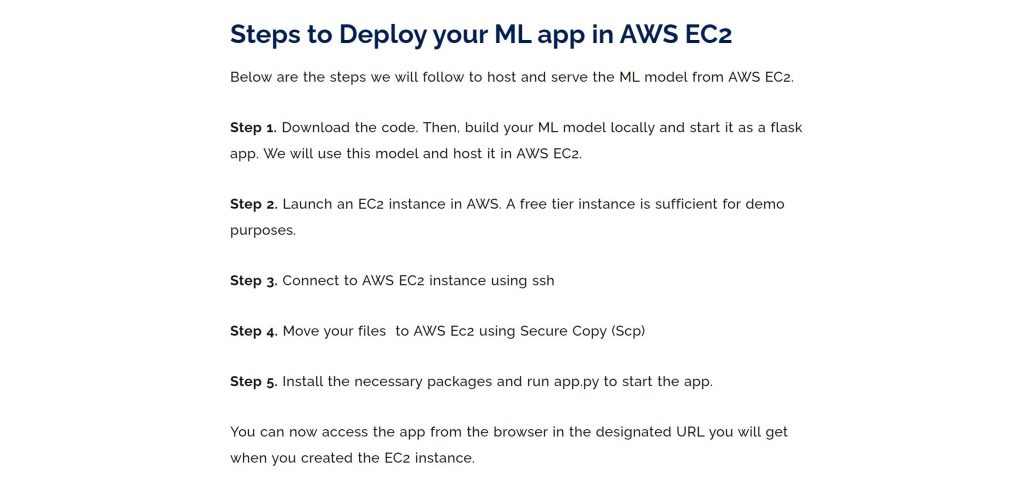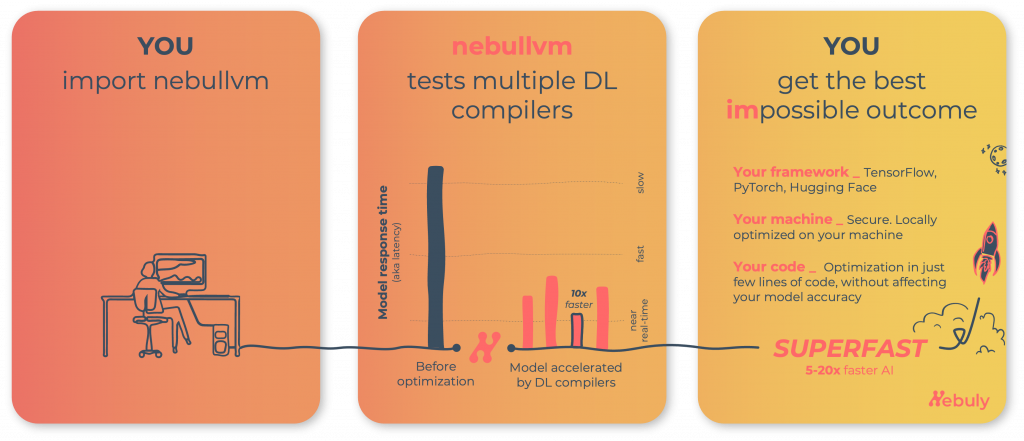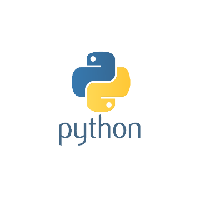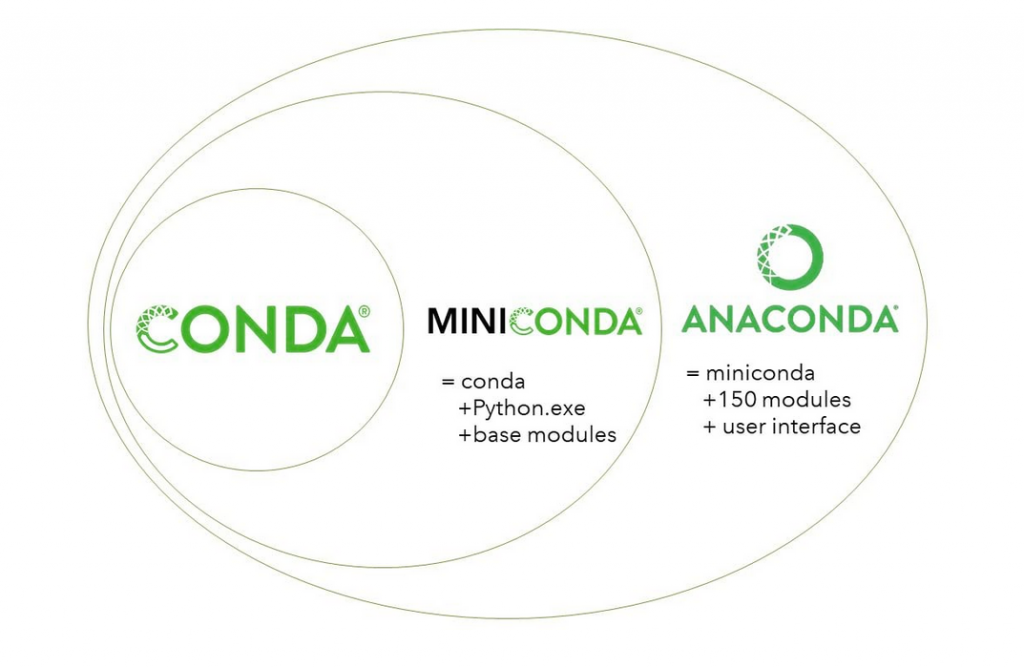## Granger Causality Test

Introduced in 1969 by Clive Granger, Granger causality test is a statistical test that is used to determine if a particular time series is helpful in forecasting another series. What is Granger Causality? Let’s first understand when is the Granger causality test is useful. Let’s say you have two time series data X and Y, …## Main Pitfalls in Machine Learning Projects

Not all Data Science projects that get kicked-off see the light of day. While this is true for any project, there are common reasons specific to Data Science projects, which can be avoided. These can either be prevented if you are aware and not commit these mistakes. Or if you see these problems, consider avoiding …## Population Stability Index (PSI)

Population stability Index (PSI) is a model monitoring metric that is used to quantify how much the distribution of a continuous response variable has changed between two given samples, typically collected at different points in time. Originally, PSI was used to check if the distribution of the Y (aka the response variable or the scoring …

## List Comprehension

Now let’s understand list comprehensions this is a slightly more advanced concept compared to list a list comprehension. Now, remember earlier, I was giving you one to one problem and in order to solve that problem you need to understand list and list comprehension. We have now understood what list is let’s get to a …

## Regression Coefficient Formula

Let’s understand the formula for the linear regression coefficients. That is the formula for both alpha and the beta. Now, if you have simple linear regression that does, you have just 1x variable in your data, you will be able to compute the values of alpha and beta using this formula. Let’s suppose you …

## Linear Regression in Machine Learning – Clearly Explained

In this lesson, I introduce what Linear regression is all about. Linear Regression is a foundational algorithm for machine learning and statistical modeling. Traditionally, Linear Regression is the very first algorithm you’d learn when getting started with predictive modeling. While there are a lot more ML and Deep learning algorithm in use today, …## Linear Regression in Machine Learning – Clearly Explained

Understanding linear regression. Let’s understand what linear regression is all about from a non-technical perspective, before we get into the details, we will first understand from a layman’s terms what linear regression is. Now, linear regression is a machine learning algorithm ml algorithm that uses data to predict a quantity of interest, typically, we call …

## Feature selection using FRUFS and VevestaX

In machine learning problems, feature selection helps in reducing overfitting, removes noisy variables, reduces memory footprint. In this article we present a new technique, namely FRUFS. The algorithm is based on the idea that the most important feature is the one that can largely represent all other features. Similarly, the second most important feature can …## Deploy ML model in AWS Ec2 – Complete no-step-missed guide

In this guide, you will understand the steps to deploy your ML model app in Amazon EC2 service. Here the ML app is a flask based. However, the same steps and ideas can be followed to host apps built with other similar applications. Note that we will not be covering how to build an ML …## Nebullvm – Tutorials and benchmarks on Nebullvm, the open-source deep learning inference accelerator

Nebullvm is an open-source library that takes a deep learning model as input and outputs an optimized version that runs 5-20 times faster on your machine. Nebullvm tests multiple deep learning compilers to identify the best possible way to execute your model on your specific hardware, without impacting the accuracy of your model (GitHub link). …

## Test banner on post## Decorators in Python – How to enhance functions without changing the code?

Decorators in python allow you to dynamically change the functionality of another function, without altering it’s code. What? Is that possible? Yes. This covers: 1. What is a decorator and how to create one? 2. Easier way to decorate functions 3. Class decorators 4. Problem with docstrings on decorated functions and how to solve. What …## Generators in Python – How to lazily return values only when needed and save memory?

Generators in python provide an efficient way of generating numbers or objects as and when needed, without having to store all the values in memory beforehand. Introduction You can think of Generators as a simple way of creating iterators without having to create a class with __iter__() and __next__() methods. So how to create a …## Iterators in Python – What are Iterators and Iterables?

Iterable in Python is any object that can be looped over. In order for an iterable to be looped over, an iterable needs to be converted to an iterator using the __iter__() method. Once created, it is actually the iterator that gets iterated over. This is also what happens internally when you run a for-loop. …## Python Module – What are modules and packages in python?

Any Python file with a .py extension is a Module in Python. A python package is a collection of such modules along with a __init__.py file. Let’s understand how to work with modules and packages with clear examples. Introduction When you work on python projects, it’s not a good practice to have all you python …## Object Oriented Programming (OOPS) in Python

Object oriented programming is an effective way of writing code. You create classes which are python objects, that represented meaningful entities which defines its own behaviour (via methods) and attributes. Let’s understand what a class is and the concepts behind Object Oriented Programming in Python Everything you have encountered so far in Python, such as …## Conda create environment and everything you need to know to manage conda virtual environment

Typical python projects uses multiple packages for various tasks. And some of the packages are shared between projects as well. Sharing same packages between projects can cause problems. How? When you update one of the packages used in a project, it might cause compatibility issues in the other packages that use it. On upgrading, it …## How to use Numpy Random Function in Python

How to use numpy.random.rand() function ? numpy.random.rand() function is used to generate random float values from an uniform distribution over [0,1). These values can be extracted as a single value or in arrays of any dimension. In this article, you will learn about various use cases of this function. Structural overview of numpy.random.rand() Syntax: numpy.random.rand(d0, …Course Preview

## Machine Learning A-Z™: Hands-On Python & R In Data Science

### Free Sample Videos:#### Machine Learning A-Z™: Hands-On Python & R In Data Science#### Machine Learning A-Z™: Hands-On Python & R In Data Science#### Machine Learning A-Z™: Hands-On Python & R In Data Science#### Machine Learning A-Z™: Hands-On Python & R In Data Science